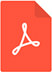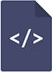# On the definition of distribution equilibrium potentials in the distribution systems with simple saltsPDF Full Text

# Abstract

A deviation in the definition of distribution equilibrium potential between electrochemical and extraction-chemical phenomena was discussed and examined quantitatively.

# Keywords

distribution equilibrium potential, inner potential, Nernst equation, conditional distribution constant, distribution ratio, distribution of simple salts, potentiometric, aqueous solutions, standard distribution, electrochemical, complicated extraction, interfaces, henderson equation, polarographic, distribution